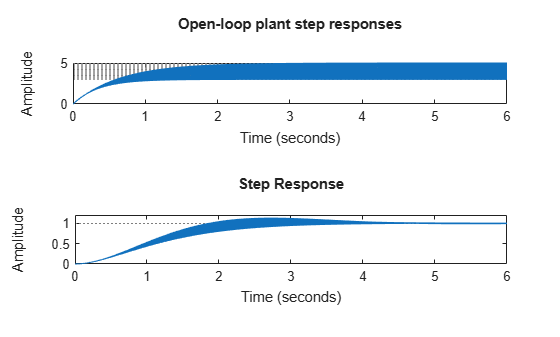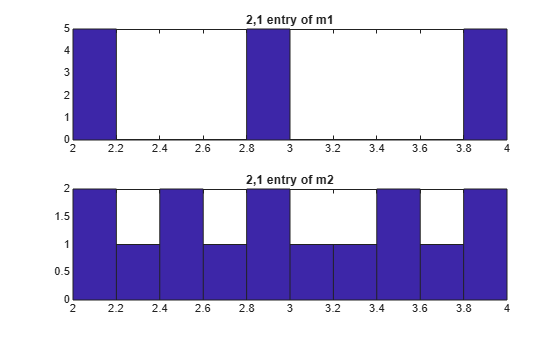# gridureal

Grid `ureal` parameters uniformly over their range

## Syntax

```B = gridureal(A,N) [B,SampleValues] = gridureal(A,N) [B,SampleValues] = gridureal(A,NAMES,N) [B,SampleValues] = gridureal(A,NAMES1,N1,NAMES2,N2,...) ```

## Description

`B = gridureal(A,N)` substitutes `N` uniformly-spaced samples of the uncertain real parameters in `A`. The samples are chosen to cut “diagonally” across the cube of real parameter uncertainty space. The array `B` has size equal to `[size(A) N]`. For example, suppose `A` has 3 uncertain real parameters, say `X`, `Y` and `Z`. Let (`x1, x2 , , and xN`) denote `N` uniform samples of `X` across its range. Similar for `Y` and `Z`. Then sample `A` at the points `(x1, y1, z1)`, `(x2, y2, z2)`, and `(xN, yN, zN)` to obtain the result `B`.

If `A` depends on additional uncertain objects, then `B` will be an uncertain object.

`[B,SampleValues] = gridureal(A,N)` additionally returns the specific sampled values (as a `structure` whose fieldnames are the names of `A'`s uncertain elements) of the uncertain reals. Hence, `B` is the same as `usubs(A,SampleValues)`.

`[B,SampleValues] = gridureal(A,NAMES,N)` samples only the uncertain reals listed in the `NAMES` variable (`cell`, or `char` array). Any entries of `NAMES` that are not elements of `A` are simply ignored. Note that `gridureal(A, fieldnames(A.Uncertainty),N)` is the same as `gridureal(A,N)`.

`[B,SampleValues] = gridureal(A,NAMES1,N1,NAMES2,N2,...)` takes `N1` samples of the uncertain real parameters listed in `NAMES1`, and `N2` samples of the uncertain real parameters listed in `NAMES2` and so on. `size(B)` will equal `[size(A) N1 N2 ...]`.

## Examples

collapse all

Create two uncertain real parameters `gamma` and `tau`. The nominal value of `gamma` is 4 and its range is 3 to 5. The nominal value of `tau` is 0.5 and its value can vary by +/- 30 percent.

```gamma = ureal('gamma',4); tau = ureal('tau',.5,'Percentage',30);```

These uncertain parameters are used to construct an uncertain transfer function `p`. An integral controller, `c`, is synthesized for the plant `p` based on the nominal values of `gamma` and `tau`. The uncertain closed-loop system `clp` is formed.

```p = tf(gamma,[tau 1]); KI = 1/(2*tau.Nominal*gamma.Nominal); c = tf(KI,[1 0]); clp = feedback(p*c,1);```

The figure below shows the open-loop unit step response (top plot) and closed-loop response (bottom plot) for a grid of 20 values of `gamma` and `tau`.

```subplot(2,1,1); step(gridureal(p,20),6) title('Open-loop plant step responses') subplot(2,1,2); step(gridureal(clp,20),6)```The plot illustrates the low-frequency closed-loop insensitivity achieved by the PI control system.

This example illustrates the different options in gridding high-dimensional (e.g., `n` greater than 2) parameter spaces.

Construct an uncertain matrix, `m`, from four uncertain real parameters, `a`, `b`, `c`, and `d`, each making up the individual entries in `m`.

```a = ureal('a',1); b = ureal('b',2); c = ureal('c',3); d = ureal('d',4); m = [a b;c d];```

First, grid the `(a,b)` space at five places, and the `(c,d)` space at three places.

`m1 = gridureal(m,{'a';'b'},5,{'c';'d'},3);`

`gridureal` evaluates the uncertain matrix `m` at these 15 grid points, resulting in the numerical matrix `m1`.

Next, grid the `(a,b,c,d)` space at 15 places.

`m2 = gridureal(m,{'a';'b';'c';'d'},15);`

`gridureal` samples the uncertain matrix `m` at these 15 points, resulting in the numerical matrix `m2`.

The (2,1) entry of `m` is just the uncertain real parameter `c`. Plot the histograms of the (2,1) entry of both `m1` and `m2`. The (2,1) entry of `m1` only takes on three distinct values, while the (2,1) entry of `m2` takes on 15 distinct values uniformly through its range.

```subplot(2,1,1) hist(squeeze(m1(2,1,:))) title('2,1 entry of m1') subplot(2,1,2) hist(squeeze(m2(2,1,:))) title('2,1 entry of m2')```